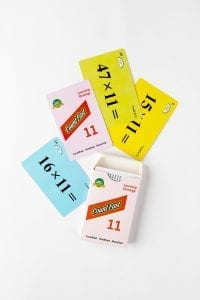# CountFast 11 Lesson Plan

####OVERVIEW & PURPOSE

Week 7 of the 3rd Grade CountFast program focuses on strategies to multiply two and three-digit numbers by 11.  Spend 15 minutes each day on one of the activities listed in this module.  Card decks should go home with students each day for additional practice with a parent at home. Each week, a new deck is introduced, and the previous deck is for the student to keep at home for continued practice.

#### EDUCATION STANDARDS

1. NCTM Standard: develop a sense of whole numbers and represent and use them in flexible ways, including relating, composing, and decomposing numbers
2. Math.Content.3.OA.B.5
Apply properties of operations as strategies to multiply and divide.

#### OBJECTIVES

1. Mentally multiply two-digit numbers by 11.
2. Mentally multiply three-digit numbers by 11.

#### MATERIALS NEEDED

1. One CountFast 11 card deck for each student.  This deck is for school and home use.  Discuss routine and expectations for taking home the deck and returning it to school each day.
2. One writing utensil per student, optional per teacher plans.

#### CountFast 11 Pack – Day 1 and 2

Teacher Model/Direct:  Use the yellow cards from the deck on Day 1 and the blue cards on Day 2.  The day one lesson is to teach students how to multiply any number by 11 when there is no need to carry over.  The day two lesson is to teach students how to multiply any number by 11 when there is a need to carry over.  Show students the following strategies: (The trick is to split the digits of the original number you are multiplying by 11 and in the middle space add those digits together to give you the answer. Remember you may need to carry the number forward)

34 x 11 = 3 (3+4) 4                                                65 x 11 = 6 (6+5) 5

= 374                                                                     carry forward = 6 (11) 5

#### Carry 1 forward and add to 6 = 715

Student Activity:  Give each student a deck and ask them to take out the yellow cards (on Day 1) or the blue cards (on Day 2).  Working with a partner, take turns holding up the cards for the partner to multiply by 11 using the strategy from the lesson.

Home Activity: Students will take home the deck and the “CountFast Home Connection” letter.  Students will review the strategy for multiplying a number by 11.  Parents can record how quickly the child multiplies all of the yellow/blue cards correctly.

#### CountFast 11 Pack – Day 3, 4, and 5

Teacher Model/Direct:  Using the same procedure as Days 1 and 2, introduce to students the mental strategy for multiplying 11 by three-digit numbers.  It is a very similar process, with an extra step.  On Day 3, use the pink cards to practice multiplication with no carry-over.  On Day 4, use the green cards to practice multiplication with carry-over.  Start by splitting the first and last digits apart and leave two spaces between.  Add the first 2 digits to fill the first space, and add the back 2 digits to fill the second space.

135 x 11 = 1 (1+3)(3+5) 5                                302 x 11 = 3 (3+0)(0+2) 2

= 1485                                                             = 3322

Student Activity:  Students should work with a partner, using the pink and green cards, to practice multiplying 11 by three-digit numbers.  If time allows, use Day 5 to practice multiplying 11 by both two-digit and three-digit numbers.

Home Activity: Students will review the strategy for multiplying 11 by a three-digit number.  Parents will use the pink and green cards with child and record how quickly the child answers all cards correctly each round.

#### Instructional Video

Stay SharpFree flash card decks to keep kids entertained and sharp!

To help you keep your kids math sharp during this crisis, we're giving you 3 free CountFast flash card decks for just \$6 in shipping.  Just have them take the quick quiz for their grade at the link below and you'll get a discount code to use on any three decks.